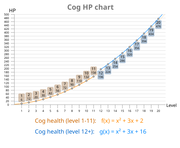## FANDOM

2,359 Pages

Guide
A guide is a helpful resource created by users on the wiki that covers a specific topic in detail and help readers gain a better understanding of the topic.
Cogs have a certain number of health points (HP) depending on their level. Cog levels range from level 1 to level 12. Level 1 Cogs have the lowest health points, while level 12 Cogs have the highest health points.

The chart below shows how much HP each Cog level has and what gag can destroy it at once.

## Health by Cog levels

Level Health Points One-hit knock gags
1 6

Cupcake (6 damage)
Flower Pot (10 damage)
Glass of Water (6 damage)

2 12

Banana Peel (12 damage)
Sandbag (18 damage)

3 20 Rake (20 damage)
4 30 Hose (30 damage)
Anvil (30 damage)
Marbles (35 damage)
5 42 Big Weight (45 damage)
Organic Whole Cream Pie (44 Damage)
Quicksand (50 damage)
6 56 Safe (60 damage)
Trap Door (70 damage)
7 72 Organic Trap Door (77 damage)
Storm Cloud (80 damage)
8 90 Opera Singer (90 damage)
Birthday Cake (100 damage)
9 110 Organic Birthday Cake (110 damage)
Organic Geyser (115 damage)
10 132 Organic Wedding Cake (132 damage)
11 156 Grand Piano (170 damage)
TNT (180 damage)
12 200 Organic Railroad (214 damage)

## FormulasA graph showing the health growth of cogs by their level. The difference in health of Level 12 Cogs can be seen

The formula for a Cog's health is the following:

$f(x) = x^{2} + 3x + 2$

Or, in a simplified way:

$f(x) = (x + 1) \times (x + 2)$

Where x is the Cog's level, and f(x) is a function that returns the health value of cogs. For instance, for Level 4 Cogs, who have a health of 30, the formula works in the following way:

$f(4) = (4 + 1) \times (4 + 2)$
$f(4) = (5) \times (6)$
$f(4) = 30$

This formula applies to every Cog level except level 12, which has 200 health points.

In order to get the cog level from a given HP, rather than getting the HP from the level, the HP formula's quadratic formula can be used (where n is the HP):

$x_{1,2} = \frac{-3 \pm \sqrt{9 - 4 * (2 - n)}}{2}$

So, for instance, in order to find which cog has 156 HP, the equation will be solved in the following way:

$x_{1,2} = \frac{-3 \pm \sqrt{9 - 4 * (2 - 156)}}{2}$
$x_{1,2} = \frac{-3 \pm \sqrt{9 - 4 * - 154}}{2}$
$x_{1,2} = \frac{-3 \pm \sqrt{9 + 616}}{2}$
$x_{1,2} = \frac{-3 \pm \sqrt{625}}{2}$
$x_{1,2} = \frac{-3 \pm 25}{2}$
$x_{1} = \frac{-3 - 25}{2} , x_{2} = \frac{-3 + 25}{2}$
$x_{1} = \frac{-28}{2} , x_{2} = \frac{22}{2}$
$x_{1} = -14 , x_{2} = 11$

Since the original equation- f(x)- is a function of a parabola, and since none are the solutions are the function's minimum value, there are two answers for the equation: -14 ,which is not a real Cog level; and 11, which does exist.

Although this formula works for all Toontown Cog levels, when going beyond regular Cog levels, this formula starts to be inapplicable in practice because of complex numbers. For any 'n' value less than -0.25, this equation introduces complex numbers, which still work in theory but not in practice.

## Trivia

• Level 12 Cogs have 200 health points, instead of the expected value from the formula- 182. This is essentially a 10% boost, rounded down. According to the formula, a Cog with 200 health should be about level 12.65 (or about -15.65, though negative values are not compatible with cog levels).
• According to the formula, the Director of Ambush Marketing would have 2,652 health, given that the Director of Ambush Marketing is level 50.
Community content is available under CC-BY-SA unless otherwise noted.